Next: One-Dimensional Fourier Optics Up: Wave Optics Previous: Multi-Slit Interference

# Thin Film Interference

In everyday life, the most common manifestation of interference occurs when light impinges on a thin film of some transparent material. For instance, the brilliant colors seen in soap bubbles, in oil films floating on puddles of water, and in the feathers of a peacock's tail, are due to this type of interference.

Suppose that a very thin film of air is trapped between two pieces of glass, as shown in Figure 70. If monochromatic light is normally (or almost normally) incident on the film then some of the light is reflected from the interface between the bottom of the upper plate and the air, and some is reflected from the interface between the air and the top of the lower plate. These two reflected light rays interfere either destructively or constructively with one another.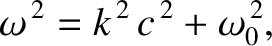Let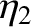be the thickness of the air film. The difference in distance traveled between the two light rays shown in the figure is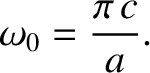. This difference introduces a phase difference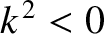between the rays. Naively, we might expect that constructive interference would occur when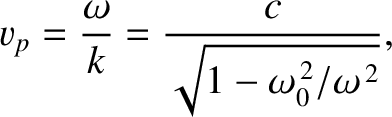, where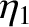is an integer, and destructive interference would occur when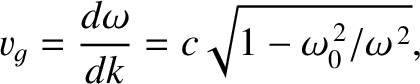. However, this is not the case, because an additional phase difference is introduced between the rays on reflection. The first ray is reflected at an interface between an optically dense medium (glass) and a less dense medium (air). There is no phase change on reflection from such an interface. (See Section 7.7.) The second ray is reflected at an interface between an optically less dense medium (air) and a dense medium (glass). There is a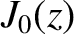radian phase change on reflection from such an interface. (See Section 7.7.) Thus, an additionalradian phase change is introduced between the two rays, which is equivalent to an additional path difference of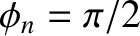. When this additional phase change is taken into account, the condition for constructive interference becomes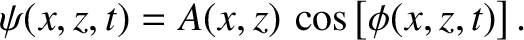(1037)

whereis an integer. Similarly, the condition for destructive interference becomes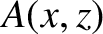(1038)

For white light, the above criteria yield constructive interference for some wavelengths, and destructive interference for others. Thus, the light reflected back from the film exhibits those colors for which constructive interference occurs.

If the thin film consists of water, oil, or some other transparent material, of refractive index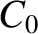then the results are basically the same as those for an air film, except that the wavelength of the light in the film is reduced from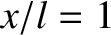(the vacuum wavelength) to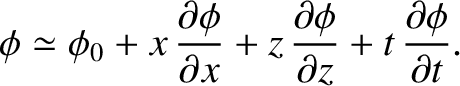. It follows that the modified criteria for constructive and destructive interference are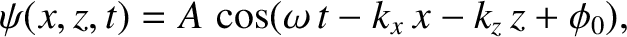(1039)

and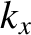(1040)

respectively.Next: One-Dimensional Fourier Optics Up: Wave Optics Previous: Multi-Slit Interference
Richard Fitzpatrick 2013-04-08